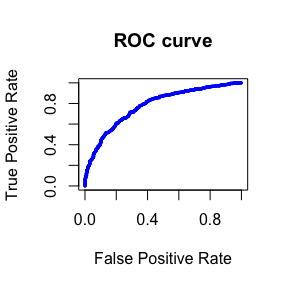# ROCaggregator use case

## Introduction

The ROCaggregator package allows you to aggregate multiple ROC (Receiver Operating Characteristic) curves. One of the scenarios where it can be helpful is in federated learning. Evaluating a model using the ROC AUC (Area Under the Curve) in a federated learning scenario will require to evaluating the model against data from different sites. This will eventually lead to partial ROCs from each site which can be aggregated to obtain a global metric to evaluate the model.

library(ROCaggregator)

## Set-up

For this use case, we’ll be using some external packages: - the ROCR to compute the ROC at each node and to validate the AUC obtained; - the pracma package to compute the AUC using the trapezoidal method; - the stats package to create a linear model;

The use case will consist of 3 nodes with horizontally partitioned data. A linear model will be trained with part of the data and tested at each node, generating a ROC curve for each.

To compute the aggregated ROC, each node will have to provide: - the ROC (consisting of the false positive rate and true positive rate); - the thresholds/cutoffs used (in the same order as the ROC); - the total number of negative labels in the dataset; - the total number of samples in the dataset;

library(ROCR)
library(pracma)
library(stats)

set.seed(13)

create_dataset <- function(n){
positive_labels <- n %/% 2
negative_labels <- n - positive_labels

y = c(rep(0, negative_labels), rep(1, positive_labels))
x1 = rnorm(n, 10, sd = 1)
x2 = c(rnorm(positive_labels, 2.5, sd = 2), rnorm(negative_labels, 2, sd = 2))
x3 = y * 0.3 + rnorm(n, 0.2, sd = 0.3)

data.frame(x1, x2, x3, y)[sample(n, n), ]
}

# Create the dataset for each node
node_1 <- create_dataset(sample(300:400, 1))
node_2 <- create_dataset(sample(300:400, 1))
node_3 <- create_dataset(sample(300:400, 1))

# Train a linear model on a subset
glm.fit <- glm(
y ~ x1 + x2 + x3,
data = rbind(node_1, node_2),
family = binomial,
)

get_roc <- function(dataset){
glm.probs <- predict(glm.fit,
newdata = dataset,
type = "response")
pred <- prediction(glm.probs, c(dataset$y)) perf <- performance(pred, "tpr", "fpr") perf_p_r <- performance(pred, "prec", "rec") list( "fpr" = perf@x.values[], "tpr" = perf@y.values[], "prec" = perf_p_r@y.values[], "thresholds" = perf@alpha.values[], "negative_count"= sum(dataset$y == 0),
"total_count" = nrow(dataset),
"auc" = performance(pred, measure = "auc")
)
}

# Predict and compute the ROC for each node
roc_node_1 <- get_roc(node_1)
roc_node_2 <- get_roc(node_2)
roc_node_3 <- get_roc(node_3)

## Aggregating the ROC from each node

Obtaining the required inputs from each node will allow us to compute the aggregated ROC and the corresponding AUC.

# Preparing the input
fpr <- list(roc_node_1$fpr, roc_node_2$fpr, roc_node_3$fpr) tpr <- list(roc_node_1$tpr, roc_node_2$tpr, roc_node_3$tpr)
thresholds <- list(
roc_node_1$thresholds, roc_node_2$thresholds, roc_node_3$thresholds) negative_count <- c( roc_node_1$negative_count, roc_node_2$negative_count, roc_node_3$negative_count)
total_count <- c(
roc_node_1$total_count, roc_node_2$total_count, roc_node_3$total_count) # Compute the global ROC curve for the model roc_aggregated <- roc_curve(fpr, tpr, thresholds, negative_count, total_count) # Calculate the AUC roc_auc <- trapz(roc_aggregated$fpr, roc_aggregated$tpr) sprintf("ROC AUC aggregated from each node's results: %f", roc_auc) #>  "ROC AUC aggregated from each node's results: 0.778901" # Calculate the precision-recall precision_recall_aggregated <- precision_recall_curve( fpr, tpr, thresholds, negative_count, total_count) # Calculate the precision-recall AUC precision_recall_auc <- -trapz( precision_recall_aggregated$recall, precision_recall_aggregated$pre) sprintf( "Precision-Recall AUC aggregated from each node's results: %f", precision_recall_auc ) #>  "Precision-Recall AUC aggregated from each node's results: 0.773897" ## Validation Using ROCR we can calculate the ROC and its AUC for the case of having all the data centrally available. The values between this and the aggregated ROC should match. roc_central_case <- get_roc(rbind(node_1, node_2, node_3)) # Validate the ROC AUC sprintf( "ROC AUC using ROCR with all the data centrally available: %f", roc_central_case$auc@y.values[]
)
#>  "ROC AUC using ROCR with all the data centrally available: 0.778901"

# Validate the precision-recall AUC
precision_recall_auc <- trapz(
roc_central_case$tpr, ifelse(is.nan(roc_central_case$prec), 1, roc_central_case$prec) ) sprintf( "Precision-Recall AUC using ROCR with all the data centrally available: %f", precision_recall_auc ) #>  "Precision-Recall AUC using ROCR with all the data centrally available: 0.773897" ## Visualization The ROC curve obtained can be visualized in the following way: plot(roc_aggregated$fpr,
roc_aggregated$tpr, main="ROC curve", xlab = "False Positive Rate", ylab = "True Positive Rate", cex=0.3, col="blue", )## Appendix ### Using pROC library Another popular package to compute ROC curves is the pROC. Similarly to the example with the ROCR package, it’s also possible to aggregate the results from ROC curves computed with the pROC package. library(pROC, warn.conflicts = FALSE) #> Type 'citation("pROC")' for a citation. get_proc <- function(dataset){ glm.probs <- predict(glm.fit, newdata = dataset, type = "response") roc_obj <- roc(c(dataset$y), c(glm.probs))
list(
"fpr" = 1 - roc_obj$specificities, "tpr" = roc_obj$sensitivities,
"thresholds" = roc_obj$thresholds, "negative_count"= sum(dataset$y == 0),
"total_count" = nrow(dataset),
"auc" = roc_obj$auc ) } roc_obj_node_1 <- get_proc(node_1) #> Setting levels: control = 0, case = 1 #> Setting direction: controls < cases roc_obj_node_2 <- get_proc(node_2) #> Setting levels: control = 0, case = 1 #> Setting direction: controls < cases roc_obj_node_3 <- get_proc(node_3) #> Setting levels: control = 0, case = 1 #> Setting direction: controls < cases # Preparing the input fpr <- list(roc_obj_node_1$fpr, roc_obj_node_2$fpr, roc_obj_node_3$fpr)
tpr <- list(roc_obj_node_1$tpr, roc_obj_node_2$tpr, roc_obj_node_3$tpr) thresholds <- list( roc_obj_node_1$thresholds, roc_obj_node_2$thresholds, roc_obj_node_3$thresholds)
negative_count <- c(
roc_obj_node_1$negative_count, roc_obj_node_2$negative_count, roc_obj_node_3$negative_count) total_count <- c( roc_obj_node_1$total_count, roc_obj_node_2$total_count, roc_obj_node_3$total_count)

# Compute the global ROC curve for the model
roc_aggregated <- roc_curve(fpr, tpr, thresholds, negative_count, total_count)

# Calculate the AUC
roc_auc <- trapz(roc_aggregated$fpr, roc_aggregated$tpr)

sprintf("ROC AUC aggregated from each node's results: %f", roc_auc)
#>  "ROC AUC aggregated from each node's results: 0.778901"

# Validate the ROC AUC
roc_central_case <- get_proc(rbind(node_1, node_2, node_3))
#> Setting levels: control = 0, case = 1
#> Setting direction: controls < cases

sprintf(
"ROC AUC using pROC with all the data centrally available: %f",
roc_central_case\$auc
)
#>  "ROC AUC using pROC with all the data centrally available: 0.778901"